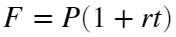# Problem 56085. Simple Interest : Calculate annual simple interest rate

Theorem : Simple Interestwhere;
• F : Future Value
• P : Present Value
• r : Annual simple interest rate (decimal)
• t : Time in years
Calculate r, given F, P and t.

### Solution Stats

94.12% Correct | 5.88% Incorrect
Last Solution submitted on Oct 04, 2023

### Community Treasure Hunt

Find the treasures in MATLAB Central and discover how the community can help you!

Start Hunting!